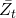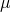Warning: "continue" targeting switch is equivalent to "break". Did you mean to use "continue 2"? in /home/calcul9/public_html/wp-content/themes/suffusion/functions/media.php on line 580

Warning: "continue" targeting switch is equivalent to "break". Did you mean to use "continue 2"? in /home/calcul9/public_html/wp-content/themes/suffusion/functions/media.php on line 583

Warning: "continue" targeting switch is equivalent to "break". Did you mean to use "continue 2"? in /home/calcul9/public_html/wp-content/themes/suffusion/functions/media.php on line 586

Warning: "continue" targeting switch is equivalent to "break". Did you mean to use "continue 2"? in /home/calcul9/public_html/wp-content/themes/suffusion/functions/media.php on line 589

Warning: "continue" targeting switch is equivalent to "break". Did you mean to use "continue 2"? in /home/calcul9/public_html/wp-content/themes/suffusion/functions/media.php on line 592
2011 June » The Calculating Investor

## Calculating the Efficient Frontier: Part 3

Jun 212011
##### Calculating the Efficient Frontier with a Risk-Free Asset

So, I’ve been feeling a little bit bogged down on this efficient frontier topic, and I’m anxious to wrap things up with this post and move on to something more original!

In the previous two posts, I have demonstrated how to calculate the efficient frontier for any number of risky assets, and I’ve shown how to calculate the portfolio weights for each point on the efficient frontier.

In this post, I will conclude this series on the efficient frontier (finally!) by demonstrating how the frontier changes with the addition of a risk-free asset.

An example Octave script is provided for calculating and plotting the efficient frontier, capital allocation line, and tangency portfolio.

##### Tangency Portfolio with a Risk-Free Asset w/return R

The tangency portfolio is the intercept point if we draw a tangent line from the risk-free rate of return (on the y-axis) to the efficient frontier for risky assets.

The equations used to calculate the portfolio weights, expected return, and variance for the tangency portfolio are shown in this section.

The variables used in these equations are described in more detail in the previous posts.  However, to review briefly,is the vector of expected returns for the risky assets, andis the covariance matrix for the returns of these assets.  The values for A, B, C, andare intermediate values calculated using the portfolio statistics and the equations for these values are shown in my initial post on this topic.   The value R is the return on the risk-free asset.  The valueis a vector showing the weight of each asset (weights sum to 1) in the tangency portfolio, andandare the expected return and variance of the tangency portfolio.## Calculating the Efficient Frontier: Part 2

Jun 142011
###### A Matrix Based Approach for Calculating Portfolio Weights

In a previous post, I showed how to calculate and plot the efficient frontier for a set of risky assets. In this post, I extend the previous example and show how to use Octave or Matlab to calculate the portfolio weights for each of the various risky assets for any point on the efficient frontier.

In my next post, I’ll conclude this series on the efficient frontier by adding a risk free asset,  showing the calculation for the tangency portfolio, and demonstrating how this creates a new frontier.

###### Calculating the Weights for Key Points on the Efficient Frontier

We can calculate the weights for any point on the efficient frontier once we know the weights for any two points on the efficient frontier.

Two easy points to calculate are the global minimum variance portfolio and the tangency portfolio for the case where the risk free rate is assumed to be zero.

Global Minimum Variance Portfolio

A point of particular interest on the efficient frontier is the “global minimum variance portfolio”.  This portfolio is the point on the efficient frontier which has the minimum variance or standard deviation.

We can solve for the mean and variance of the global minimum variance portfolio by setting the derivative of the equation for the variance to zero and solving for.  The equations for calculating the A,B,C andvalues are given in the previous post.Setting the derivative with respect toto zero gives:Running through all the algebra gives:Finally, the portfolio weights for the global minimum variance portfolio are given by:## Calculating the Efficient Frontier: Part 1

Jun 072011
###### A Matrix Based Example of  Mean-Variance Optimization using Octave

The concept of  an “efficient frontier” was developed by Harry Markowitz in the 1950s.  The efficient frontier shows us the minimum risk (i.e. standard deviation) that can be achieved at each level of expected return for a given set of risky securities.

Of course, to calculate the efficient frontier, we need to have an estimate of the expected returns and the covariance matrix for the set of risky securities which will used to build the optimal portfolio.  These parameters are difficult (impossible) to forecast, and the optimal portfolio calculation is extremely sensitive to these parameters.  For this reason, an efficient frontier based portfolio is difficult to successfully implement in practice.  However, a familiarity with the concept is still very useful and will help to develop intuition about diversification and the relationship between risk and return.

###### Calculating the Efficient Frontier

In this post, I’ll demonstrate how to calculate and plot the efficient frontier using the expected returns and covariance matrix for a set of securities.

In a future post, I’ll demonstrate how to calculate the security weights for various points on this efficient frontier using the two-fund separation theorem.

###### Calculations

In order to calculate the efficient frontier using n assets, we need two inputs.  First, we need the expected returns of each asset.  The vector of expected returns will be designated.  The second input is the variance-covariance matrix for the n assets.  This covariance matrix will be designated as.  We also need a unity vector () with the same length as the vector.

Once we have this information, we can run the following calculations using a matrix based mathematical program such as Octave or Matlab.